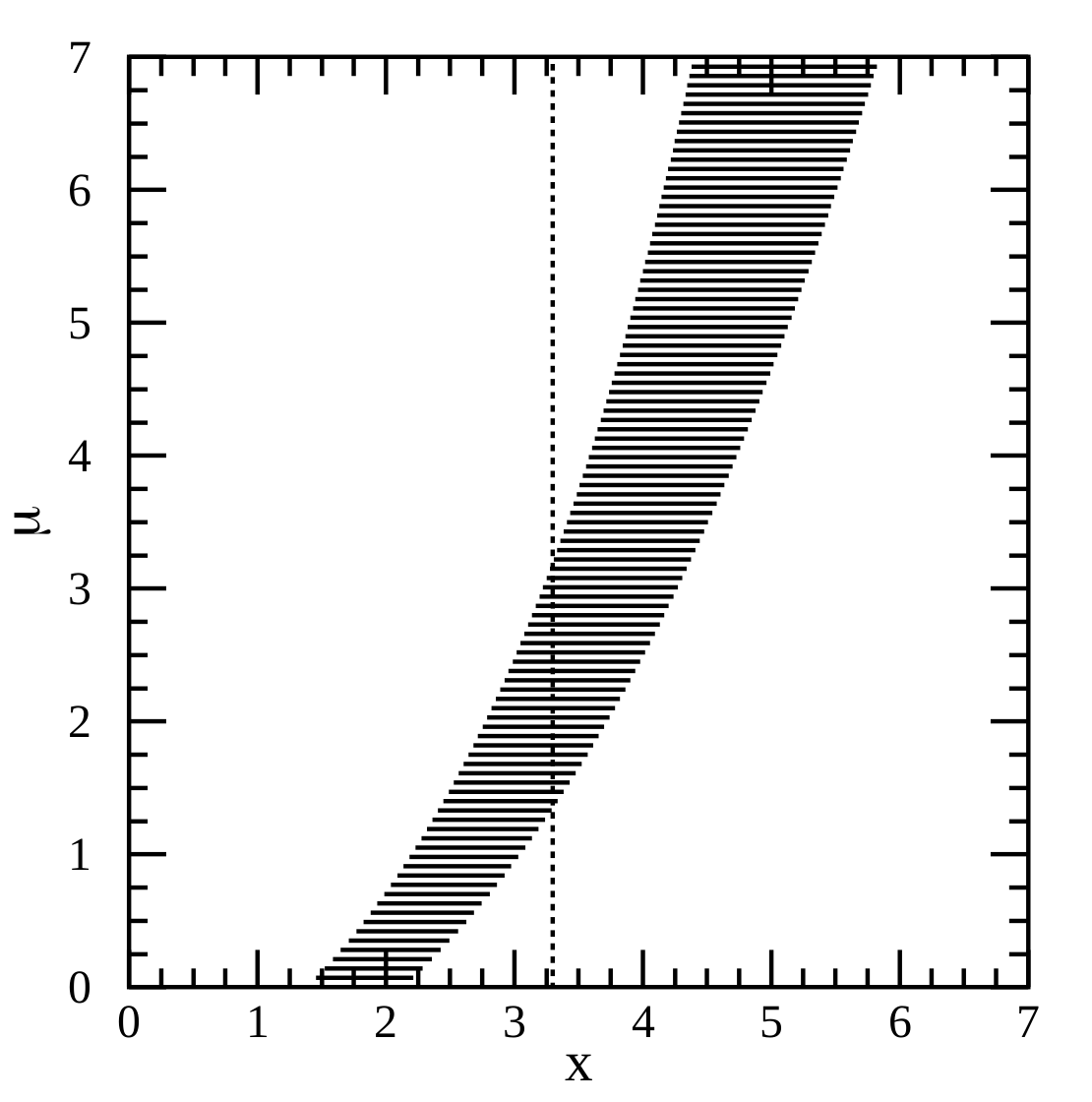# Moments for a bivariate beta distribution

A common choice for a probability distribution of a probability is the beta distribution. It has the required support between 0 and 1, and with its two parameters we can obtain a pretty wide qualitative range for the probability density function. What should we do if we want to create correlated probabilities? We might look for some kind of multivariate generalisation of the beta distribution, … Continue reading Moments for a bivariate beta distribution# The method of confidence belts illustrated

What is a confidence interval, really? We all learnt in undergrad how to find CIs for a standard distribution, but plugging numbers into equations never gave me a deep intuition for what was really going on. A worded definition is probably more helpful. Paraphrasing a bit from Wikipedia, we can think of the meaning of the confidence interval in terms of the procedure that we … Continue reading The method of confidence belts illustrated

# Estimating undetected extinctions

The purpose of this blog post is to give a simplified account of how the Chisholm et al. (2016) method works for estimating undetected extinctions. To estimate the historical extinction rate within a taxonomic group, a naive approach would be to divide the number of species known to be extinct by the total number of species. However, this does not account for the historical process … Continue reading Estimating undetected extinctions

# Some notes on the Principle of Indifference

A classical statement of the Principle of Indifference (PI) is as follows (p. 45 Keynes, 1921): if there is no known reason for predicating of our subject one rather than another of several alternatives, then relatively to such knowledge the assertions of each of these alternatives have an equal probability. Thus equal probabilities must be assigned to each of several arguments, if there is an … Continue reading Some notes on the Principle of Indifference

# The Principle of Indifference is actually two principles in one

In a previous post, I wrote about the philosophical problems caused by the Principle of Indifference. The problems are illustrated with a variety of thought-experiments that create paradoxes, such as Bertrand’s paradox. I also discussed how a problem in ecological modelling for conservation decision-making seems closely related to this philosophical problem. When I realised this connection, it seemed to me that, in order to solve … Continue reading The Principle of Indifference is actually two principles in one